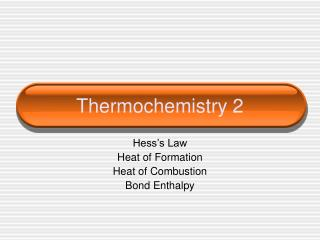Download PresentationThermochemistry 2

# Thermochemistry 2 - PowerPoint PPT Presentation

Thermochemistry 2. Hess’s Law Heat of Formation Heat of Combustion Bond Enthalpy. Hess’s Law. Want: C(graphite)  C(diamond) H = ? Given: C(graphite) + O2  CO2 H = -394 KJ C (diamond) + O2  CO2 H = -396 KJ. Hess’s Law. Want: 2B(s) + 3H 2 (g)  B 2 O 3 (g) H = ?I am the owner, or an agent authorized to act on behalf of the owner, of the copyrighted work described.
Download Presentation## Thermochemistry 2

An Image/Link below is provided (as is) to download presentation

Download Policy: Content on the Website is provided to you AS IS for your information and personal use and may not be sold / licensed / shared on other websites without getting consent from its author.While downloading, if for some reason you are not able to download a presentation, the publisher may have deleted the file from their server.

- - - - - - - - - - - - - - - - - - - - - - - - - - E N D - - - - - - - - - - - - - - - - - - - - - - - - - -
Presentation Transcript
1. Thermochemistry 2 Hess’s Law Heat of Formation Heat of Combustion Bond Enthalpy

2. Hess’s Law Want: C(graphite)  C(diamond) H = ? Given: C(graphite) + O2  CO2 H = -394 KJ C (diamond) + O2  CO2 H = -396 KJ

3. Hess’s Law Want: 2B(s) + 3H2 (g)  B2O3(g) H = ? Given: 2B (s) + 3/2 O2 (g)  B2O3(s) H = -1273 KJ B2H6 (g) + 3O2 (g)  B2O3 (s)+ 3H2O (g)H = -2035 KJ H2(g) + ½ O2 (g)  H2O (l) H = -286 KJ H2O(l)  H2O(g) H = 44KJ

4. Standard Enthalpy of Formation (Hf) • Standard Enthalpy of ____________ - enthalpy change when one mole of a substance is formed from its elements, in their standard states, under standard conditions.

5. Standard Enthalpy of Formation (Hf) • Write equations to represent the following processes. • The standard enthalpy of formation of CH3Br • The standard enthalpy of formation of CH3COC2H5 • The standard enthalpy of formation of CaCO3

6. Standard Enthalpy of Formation (Hf) • What is the Hf for CuS (s)? • Look up in Appendix • What is the Hf for 2 moles of FeCl3 (s)? • Remember, these are heats of FORMATION which means that the compound is being produced. If it on the reactant side, you must flip the sign!

7. Standard Enthalpy of Formation (Hc) • Standard Enthalpy of ____________ - enthalpy change when one mole of a substance is completely burned in oxygen under standard conditions. • Energy is usually released in such a reaction Hc, so it will usually be negative.

8. Standard Enthalpy of Formation (Hc) • Write the reaction for the following… • Hc [C2H6(g)] = - 1565 kJ/mol • The standard enthalpy of combustion of CH4 (g) • The standard enthalpy of combustion of Al(s)

9. Example 1 (do not use Appendix) • Calculate the standard enthalpy of formation of ethane (C2H6), given the following combustion data… • C(graphite) = -393 kJ/mol • H2(g) = -286 kJ/mol • C2H6(g) = -1560 kJ/mol

10. Example 2 (do not use appendix) • Calculate the standard enthalpy of combustion of propan-2-ol (CH3CH(OH)CH3), given the following data • Enthalpies of combustion for C(graphite) = -393 kJ/mol and H2(g) = -286 kJ/mol. • Enthalpy of formation of propan-2-ol = -318 kJ/mol

11. Examples • Calculate the H for the following reaction using the appendix • C3H8 (g) + 5O2 (g)  3CO2 (g) + 4H2O (l) • Is the reaction endothermic or exothermic? Why?

12. Examples • How much heat will be released from the combustion of 1.80 g of C6H6. Use the heat of formation data in the appendix.

13. Example • The Thermite reaction can be used to produce molten iron for welding railway tracks together. • Fe2O3(s) + 2Al(s) Al2O3(s) + 2Fe(s) • Calculate the enthalpy change in the Thermite reaction, given the standard enthalpies of formation of iron (III) oxide and aluminum oxide are -823 and -1675 kJmol-1, respectively.

14. Bond Enthalpies • The strength of the bond in a diatomic covalent molecule is given by the bond dissociation energy. • For example hydrogen, H2 or H-H • H2(g)  2H(g) BDE= +436 kJ

15. Bond Enthalpies • In order to ____________ a bond, energy must be put in (____________ process) • When ____________ a bond, energy is released (____________ process).

16. Bond Enthalpy Example 1 • Calculate the standard enthalpy of the reaction below. • CH3CH=CH2 + H2 CH3CH2CH3

17. Bond Energy Data

18. Bond Enthalpy Example 2 • Calculate the enthalpy change for the reaction below. • CH3CH=CH2 + Br2 CH2BrCHBrCH3

19. Bond Energy Data

20. Phase Diagrams • An area on a phase diagram represents one ____________ , a line represents the conditions under which two phases can exist in ____________ . • The ____________ point describes the conditions under which all three phases can coexist. • The ____________ point describes the maximum temperature that a liquid of the substance can exist. • Above this temperature the difference between the liquid and the gas disappear and the substance is referred to as a ____________ .

21. Phase Diagrams

22. Heating Curve